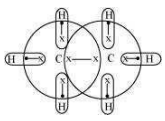For carbon hydrides of type ${C}_{n}{H}_{2n+2}$ , the following hydrides are possible for

For a hydride to act as a Lewis acid i.e., electron accepting, it should be electrondeficient. Also, for it to act as a Lewis base i.e., electron donating, it should be electronrich. Taking ${C}_{2}{H}_{6}$ as an example, the total number of electrons are 14 and the total covalent bonds are seven. Hence, the bonds are regular 2e– -2 centered bonds.Hence, hydride ${C}_{2}{H}_{6}$  has sufficient electrons to be represented by a conventional Lewis structure. Therefore, it is an electron-precise hydride, having all atoms with complete octets. Thus, it can neither donate nor accept electrons to act as a Lewis acid or Lewis base.